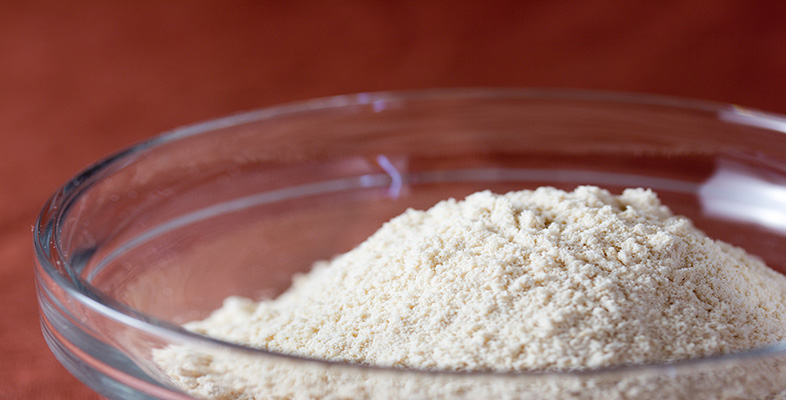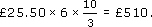Rounding and estimation

This free course is available to start right now. Review the full course description and key learning outcomes and create an account and enrol if you want a free statement of participation.

Free course

# 3.3.1 Try some yourself

## Activity 22

A friend has been quoted a price of £25.50 per square yard for tarmac surfacing of his yard. The yard measures 6 yards by 10 feet. Here is his calculation of the total cost. What is wrong with it?

cost = £25.50 × 6 × 10 = £1530

He has not used the same units. The figure 10 he used refers to feet, so in a calculation using yards he needs 10/3 (3 feet = 1 yard). The cost should be## Activity 23

Another friend, Kim, wishes to reproof her tent. A litre tin of ‘proofing agent’ states that the coverage is roughly 5 square metres per litre. The manufacturer's measurements of the tent, which is a ridge tent, are: height 2 m, length 3 m. However, Kim realises that she needs the other measurements. Here is her diagram and calculation.

The problem: how many litres of proofing agent to buy to cover the tent?

Area of 2 side panels 2 × 3 × 250 = 1500

Area of end door panels together 2 × 150 + 150 = 450

Total area = 1950

Number of litres = 1950 ÷ 5 = 390

Kim thinks this is rather a lot and asks you to help. Find her errors.

The errors are

1. Not converting the centimetres to metres.

2. The order of operations for calculating the end door panels.

Areas are:

sides 2 × 3 × 2.5 = 15 metres squared

doors 2 × (1.5 + 1.5) = 6 metres squared

Total area = 21 metres squared

Number of litres = 21 ÷ 5 = 4.2 litres

Therefore Kim needs to buy 5 litres of proofing agent.

MU120_4M2